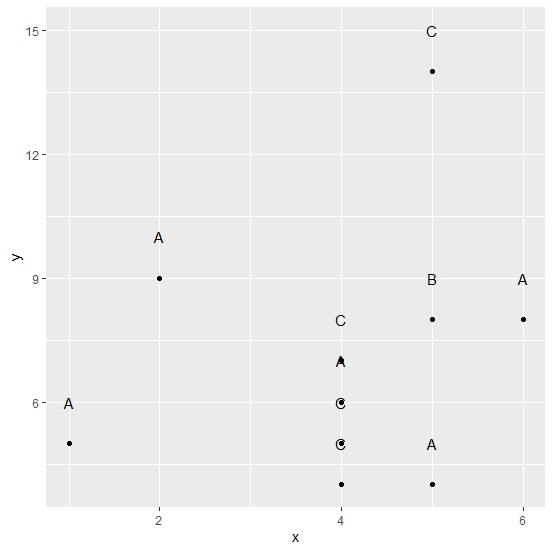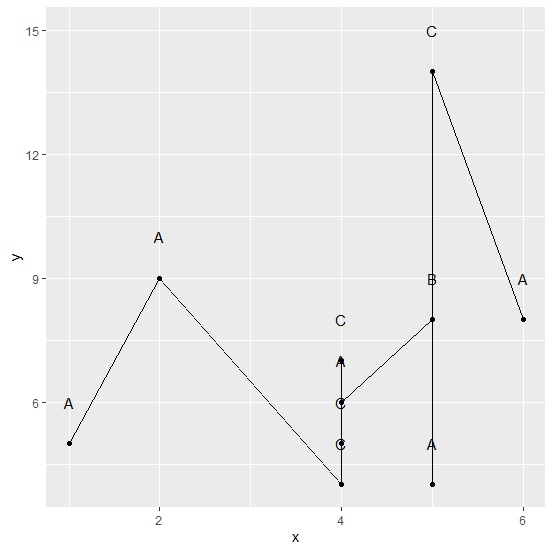# How to join points in a point chart with lines using ggplot2 in R?

Usually, a point chart is created to assess the relationship or movement of two variables together but sometimes these points are scattered in a way that makes confusion. Hence, data analyst or researcher try to visualize this type of graph by joining the points with lines. In ggplot2, this joining can be done by using geom_line() function.

Consider the below data frame −

## Example

Live Demo

set.seed(111)
x<-rpois(10,5)
y<-rpois(10,8)
grp<-sample(LETTERS[1:3],10,replace=TRUE)
df<-data.frame(x,y,grp)
df

## Output

  x  y grp
1 5 8  B
2 6 8  A
3 4 4  B
4 5 4  B
5 4 5  C
6 4 7  A
7 1 5  B
8 5 14 A
9 4 6  C
10 2 9 B

Loading ggplot2 package and creating a point chart between x and y −

## Example

library(ggplot2)
ggplot(df,aes(x,y))+geom_point()+geom_text(data=df,aes(x=x,y=y+1,label=grp))

## OutputCreating point chart between x and y with lines joining the points −

## Example

ggplot(df,aes(x,y))+geom_point()+geom_line()+geom_text(data=df,aes(x=x,y=y+1,label=grp))

## Output